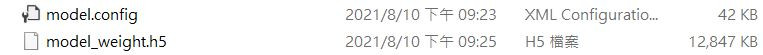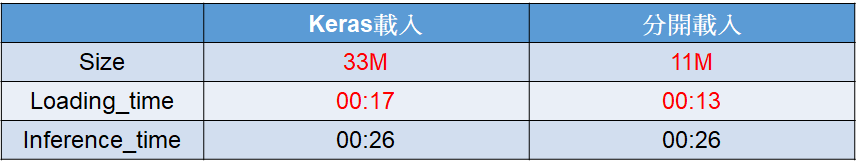#DAY 20
0
AI & Data

## DAY29 Aidea專案實作-AOI瑕疵檢測(4/4)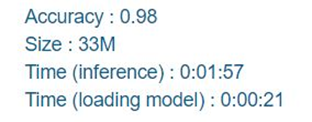## 設置動態學習率

``````#動態學習率
from tensorflow.keras.callbacks import ReduceLROnPlateau
LR_function=ReduceLROnPlateau(monitor='val_acc',
patience=5,
# 5 epochs 內acc沒下降就要調整LR
verbose=1,
factor=0.5,
# LR降為0.5
min_lr=0.00001
# 最小 LR 到0.00001就不再下降
)
``````

``````callbacks_list = [LR_function]
``````

## 神經網路剪枝(Pruning)

### 先下載所需套件

``````pip install -q tensorflow-model-optimization
``````
``````import tensorflow_model_optimization as tfmot
``````

### 設定剪掉0.5-0.8的神經元

``````prune_low_magnitude = tfmot.sparsity.keras.prune_low_magnitude

batch_size = 16
epochs = 3
validation_split = 0.2

num_images = train.shape * (1 - validation_split)
end_step = np.ceil(num_images / batch_size).astype(np.int32) * epochs
``````
``````# Define model for pruning.
pruning_params = {
'pruning_schedule': tfmot.sparsity.keras.PolynomialDecay(initial_sparsity=0.50,
final_sparsity=0.80,
begin_step=0,
end_step=end_step)
}
``````

### 一樣使用MobileNet

``````model=tf.keras.applications.MobileNet(weights='imagenet',input_shape=(128, 128, 3), include_top=False)
x = layers.GlobalAveragePooling2D()(model.output)
outputs = layers.Dense(6, activation="softmax")(x)
model=Model(inputs=model.inputs,outputs=outputs)
``````
``````#Pruning
model_for_pruning = prune_low_magnitude(model, **pruning_params)
``````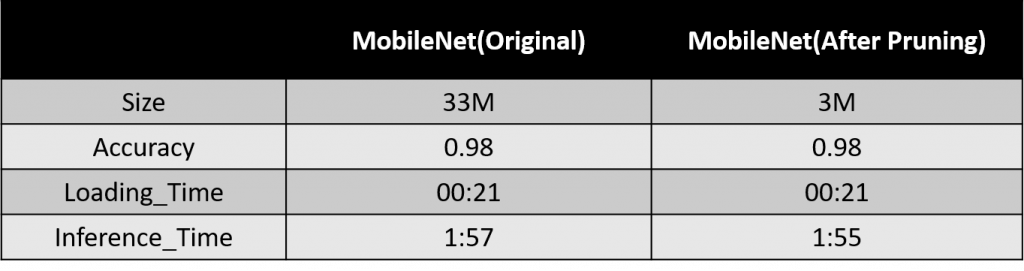## 改變預測方式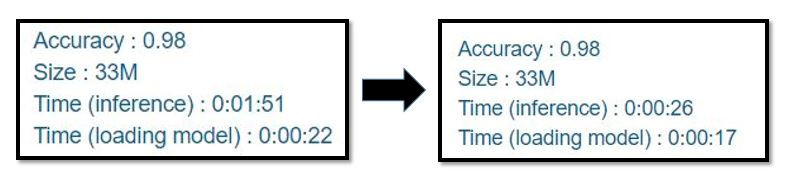## 模型儲存方式

``````#儲存架構
from keras.models import model_from_json
json_string = model.to_json()
with open("model.config", "w") as text_file:
text_file.write(json_string)

#儲存權重
model.save_weights("model_weight.h5")
``````